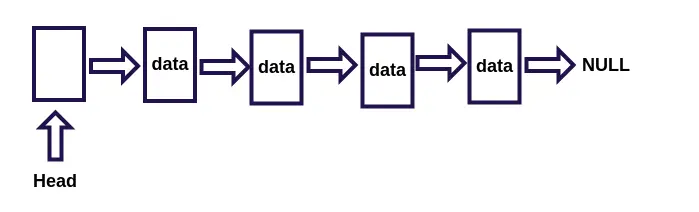``````for (i = 0; i < this.length - 1; i++) {
this.dataStore[i] = this.dataStore[i + 1];
}
````````````Node，单个节点
- element，节点数据
- next，下一个节点的指针

- insert，插入节点
- find，查找一个节点
- remove，删除一个节点
- findPrevious，查找节点的前驱节点
- print，打印链表
``````

## JavaScript 完整描述

``````function Node(element) {
this.element = element;
this.next = null;
}
}
insert: function (target, element) {
var newNode = new Node(target);
var current = this.find(element);
newNode.next = current.next;
current.next = newNode;
return this;
},
find: function (target) {
while (currentNode.element !== target) {
currentNode = currentNode.next;
}
return currentNode;
},
remove: function (element) {
var preNode = this.findPrevious(element);
if (preNode.next !== null) {
preNode.next = preNode.next.next;
return true;
}
return false;
},
findPrevious: function (element) {
while (current.next !== null && current.next.element !== element) {
current = current.next;
}
return current;
},
print: function () {
var str = '';
while (current) {
str += current.element + '->';
current = current.next;
}
console.log(str.substr(0, str.length - 2));
},
};
``````

## 分析

### 插入节点

1. 通过 find 方法找到要插入的节点位置 target
2. 创建新的节点
3. 当前节点的 next 指向 target 的 next
4. target.next 指向新节点### 删除节点

1. 找到要删除元素的前驱 preNode
2. 将 preNode 的下一个节点指向 preNode 的下一个节点(要删除的节点)的下一个节点

## 测试

``````var list = new LinkList();
list